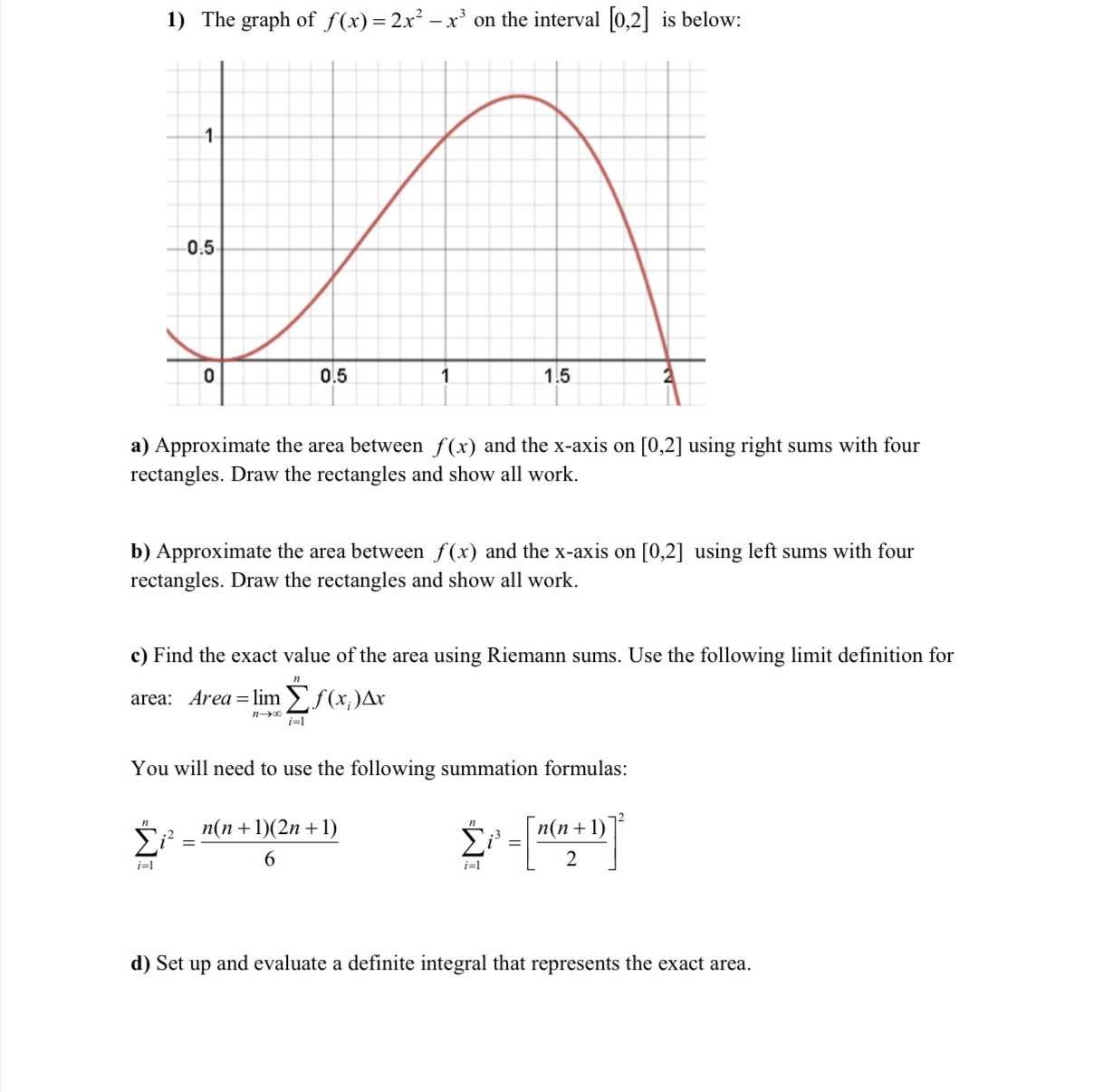### ¿Todavía tienes preguntas de matemáticas?

Pregunte a nuestros tutores expertos
Algebra
Pregunta1) The graph of $$f ( x ) = 2 x ^ { 2 } - x ^ { 3 }$$ on the interval $$[ 0,2 ]$$ is below:

a) Approximate the area between $$f ( x )$$ and the $$x$$ -axis on $$[ 0,2 ]$$ using right sums with four rectangles. Draw the rectangles and show all work.

b) Approximate the area between $$f ( x )$$ and the x-axis on $$[ 0,2 ]$$ using left sums with four rectangles. Draw the rectangles and show all work.

c) Find the exact value of the area using Riemann sums. Use the following limit definition for area: Area $$= \lim _ { n \rightarrow \infty } \sum _ { i = 1 } ^ { n } f ( x _ { i } ) \Delta x$$

You will need to use the following summation formulas:

$$\sum _ { i = 1 } ^ { n } i ^ { 2 } = \frac { n ( n + 1 ) ( 2 n + 1 ) } { 6 } \sum _ { i = 1 } ^ { n } i ^ { 3 } = [ \frac { n ( n + 1 ) } { 2 } ] ^ { 2 }$$

d) Set up and evaluate a definite integral that represents the exact area.

Area under the curve $$f ( x ) = ( 2 x ^ { 2 } - x ^ { 3 } )$$ on interval $$[ 0,2 ] : \frac { 4 } { 3 }$$